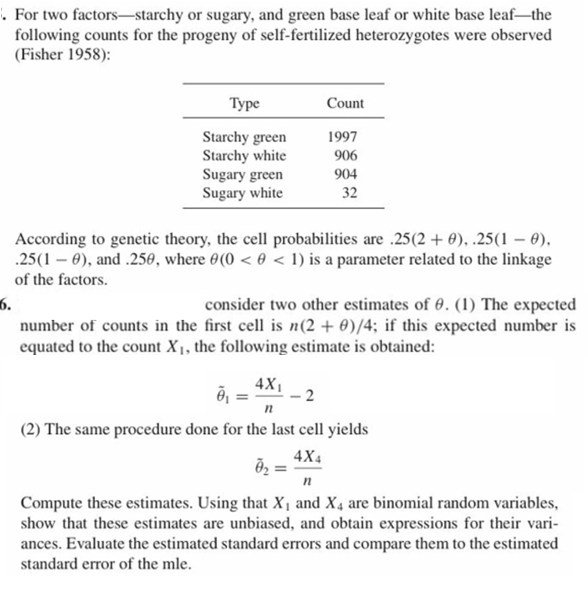# . For two factors-starchy or sugary, and green base leaf or white base leaf-thefollowing counts for the progeny of self-fertilized heterozygotes were observed(Fisher 1958):ТуреCountStarchy greenStarchy whiteSugary greenSugary white199790690432According to genetic theory, the cell probabilities are .25(2 + 0), .25(1 - 0),25(1 ), and .2560, where 0(0 < 0

Question
9 viewshelp_outlineImage Transcriptionclose. For two factors-starchy or sugary, and green base leaf or white base leaf-the following counts for the progeny of self-fertilized heterozygotes were observed (Fisher 1958): Туре Count Starchy green Starchy white Sugary green Sugary white 1997 906 904 32 According to genetic theory, the cell probabilities are .25(2 + 0), .25(1 - 0), 25(1 ), and .2560, where 0(0 < 0<1) is a parameter related to the linkage of the factors. 6. number of counts in the first cell is n(2 0)/4; if this expected number is equated to the count X1, the following estimate is obtained: consider two other estimates of 0. (1) The expected 4X1 п (2) The same procedure done for the last cell yields 4X4 n Compute these estimates. Using that X and X4 are binomial random variables show that these estimates are unbiased, and obtain expressions for their vari- ances. Evaluate the estimated standard errors and compare them to the estimated standard error of the mle fullscreen
check_circle

Step 1

Introduction:

Denote X1X2X3 and X4 as the counts for the progeny of self-fertilized heterozygotes- starchy green (1), starchy white (2), sugary green (3), and sugary white (4) respectively.

The probability distribution of the four types of progenies can be expressed as follows:

Step 2

MLE calculation can be done as shown below.

Step 3

theta-bar = ...

### Want to see the full answer?

See Solution

#### Want to see this answer and more?

Solutions are written by subject experts who are available 24/7. Questions are typically answered within 1 hour.*

See Solution
*Response times may vary by subject and question.
Tagged in

### Statistics